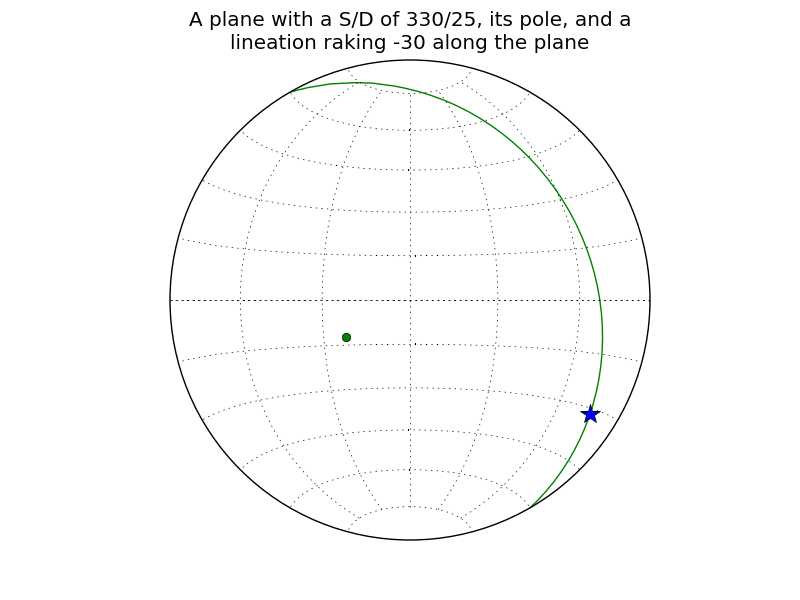# Lambert transform plot for hemisphere

First off, my apologies for resurrecting a 2-month old post…

I’m playing around with plotting with the Lambert transform. I want to
only plot one hemisphere at a time.

I happened to be doing exactly this recently, so I thought I’d post my approach to it.

from matplotlib.transforms import Affine2D

from matplotlib.projections import LambertAxes, register_projection

class LambertHemisphereAxes(LambertAxes):

``````name = 'lambert_hemisphere'
def __init__(self, *args, **kwargs):

self.horizon = kwargs.pop('horizon', 90)

LambertAxes.__init__(self, *args, **kwargs)

def _get_affine_transform(self):

transform = self._get_core_transform(1)
xscale, _ = transform.transform_point((self.horizon, 0))

_, yscale = transform.transform_point((0, np.pi / 2.0))
return Affine2D() \

.scale(0.5 / xscale, 0.5 / yscale) \
.translate(0.5, 0.5)
``````

register_projection(LambertHemisphereAxes)

Plotting with a Lambert project for only one hemisphere should then be as simple as:

ax = plt.subplot(111, projection=‘lambert_hemisphere’)

I’ve attached a quick example, just to show the general idea.

Hope that helps,
-Joe···

On Tue, 02 Mar 2010 at 3:23pm Angus McMorland wrote:

On Tue, 02 Mar 2010 at 3:23pm Angus McMorland wrote:

Hi all,

I’m playing around with plotting with the Lambert transform. I want to
only plot one hemisphere at a time.

I see that if I create the appropriate axes:

ax = plt.subplot(111, projection=‘lambert’)

the resulting axis object’s ax.get_xlim() and ax.get_ylim() methods
give the limits in rads of the plot, but the corresponding
ax.set_xlim(), and ax.set_ylim() functions don’t do anything. Is there
an alternative way to achieve my goal?

Many thanks,

## Angus.

AJC McMorland
Post-doctoral research fellow
Neurobiology, University of Pittsburgh